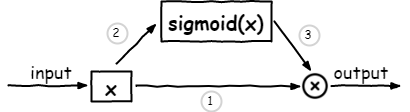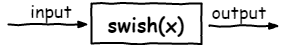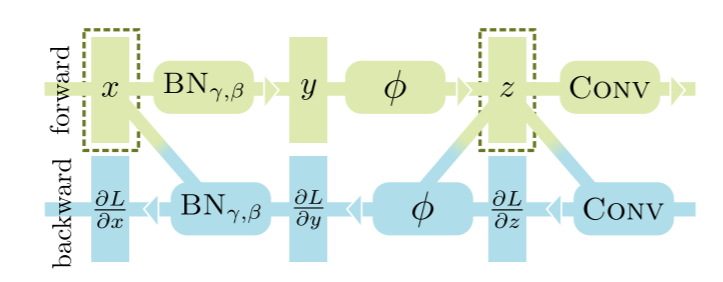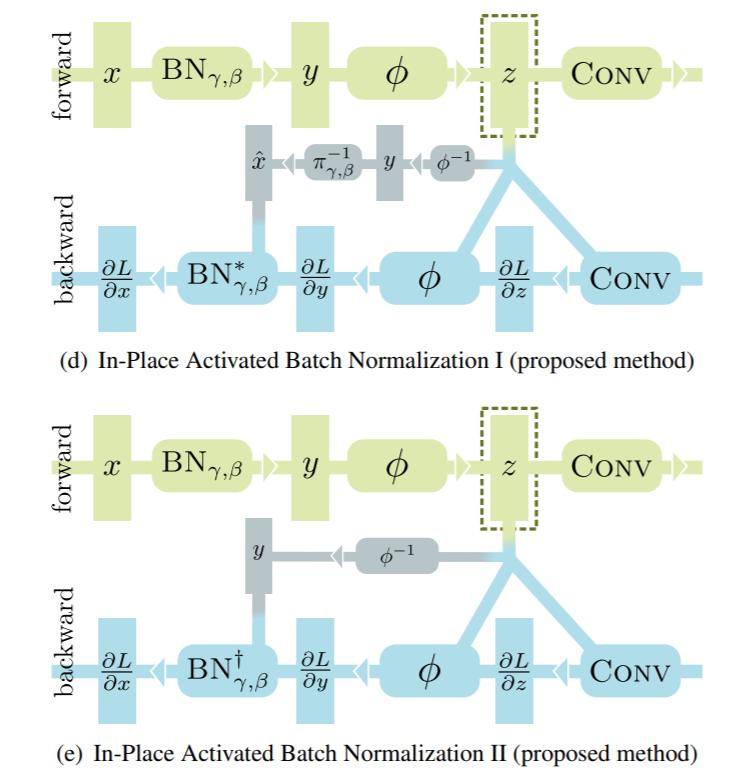bindog study Machine Learning & Security

2020-05-20

## 反向传播是如何工作的？f(x, y) = x * y
g(x) = sigmoid(x)  # 1 / (1 + exp(-x))
# gradient for x: sigmoid(x) * (1 - sigmoid(x))


## 显存被谁吃掉了

• 在适用乘法的求导规则时，要求我们要事先保留下中间结果x和sigmoid(x)，有人可能会说只保留一个x不就可以了吗？sigmoid(x)可以通过计算得出，注意框架定义的乘法及其求导规则是通用规则，乘法的左右两边完全可能是不相关的两个值，所以必须同时保留下来。

• 在对sigmoid函数适用求导规则时，需要存下中间结果x

## 手动合并OP

swish(x) = x * sigmoid(x)
# gradient for x: sigmoid(x) + x * sigmoid(x) * (1 - sigmoid(x))x的梯度可以直接根据新的规则求出，而在新的规则下，我们只需要保留x这一个中间结果即可，sigmoid(x)可以根据x求出。## 还能更进一步吗？

class CheckpointFunction(torch.autograd.Function):

@staticmethod
def forward(ctx, run_function, preserve_rng_state, *args):
check_backward_validity(args)
ctx.run_function = run_function
ctx.preserve_rng_state = preserve_rng_state
if preserve_rng_state:
ctx.fwd_cpu_state = torch.get_rng_state()
# Don't eagerly initialize the cuda context by accident.
# (If the user intends that the context is initialized later, within their
# run_function, we SHOULD actually stash the cuda state here.  Unfortunately,
# we have no way to anticipate this will happen before we run the function.)
if torch.cuda._initialized:
ctx.fwd_gpu_devices, ctx.fwd_gpu_states = get_device_states(*args)
ctx.save_for_backward(*args)
outputs = run_function(*args)
return outputs

@staticmethod
def backward(ctx, *args):
raise RuntimeError("Checkpointing is not compatible with .grad(), please use .backward() if possible")
inputs = ctx.saved_tensors
# Stash the surrounding rng state, and mimic the state that was
# present at this time during forward.  Restore the surrounding state
# when we're done.
rng_devices = []
rng_devices = ctx.fwd_gpu_devices
with torch.random.fork_rng(devices=rng_devices, enabled=ctx.preserve_rng_state):
if ctx.preserve_rng_state:
torch.set_rng_state(ctx.fwd_cpu_state)
set_device_states(ctx.fwd_gpu_devices, ctx.fwd_gpu_states)
detached_inputs = detach_variable(inputs)
outputs = ctx.run_function(*detached_inputs)

if isinstance(outputs, torch.Tensor):
outputs = (outputs,)
for inp in detached_inputs)


## CUDA版的swish activation

pytorch在用户自定义扩展上也是做了非常多的支持，用户能非常方便的使用pytorch底层定义好的一些类和函数；在写CUDA函数时，pytorch还提供了一个CUDAApplyUtils.cuh头文件，专门用于优化pointwise操作的情况，以减小拷贝和临时存储的显存浪费（用于lambda函数，函数名非常直观，CUDA_tensor_applyN表示操作数的个数，N可以为1,2,3,4，用户还可以指定每个操作数的属性，如只读/读写，针对每对情形都有专门的优化实现）

• 无优化纯Python：GPU memory=6383MB，time=223ms
• 合并算子(Python)：GPU memory=5139MB，time=234ms
• 合并算子(CUDA)：GPU memory=5143MB，time=188ms